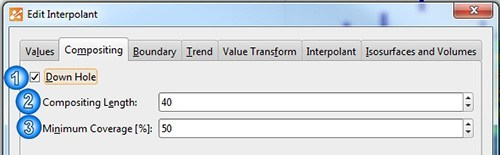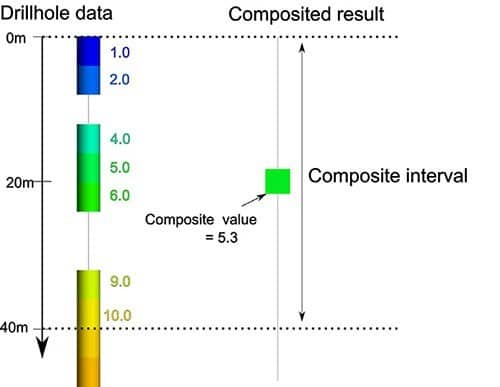## Compositing numeric and assay data

Leapfrog Geo allows users to easily manipulate drilling data.This blog, written by Applications Specialist Kirk Spragg, explains the process of compositing numeric data. Numeric data compositing takes numeric data that is unevenly spaced down drillholes, and turns it into data that is regularly spaced down the same drillholes. In this article, we explain the separate stages of the compositing process in detail. We also show you how Leapfrog Geo’s compositing algorithm works.

## The compositing process

The compositing process is performed in three stages:

1. Numeric data down drillholes is imported and desurveyed by Leapfrog Geo.
2. The user configures replacement rules for invalid and special values in the drillhole data. These rules are applied to transform invalid and special values into numeric values.
3. The resulting numeric data is composited to regularly spaced points down drillholes.

This three stage process is shown in Figure 1 below.

### Stage 1: Processing the data

First drillhole data is imported into Leapfrog Geo. Usually this data contains some invalid and/or special values. These values are transformed into numeric values in stage 2 where the user can define actions to replace the invalid or special values with numeric values. Alternatively, the user can specify certain values that are to be omitted from further processing. These actions are then applied to the drillhole data – all further processing will make use of the processed data. In the final stage, this data is composited into regularly spaced points down the drillholes. The user controls how these points are spaced and how they are generated by setting a compositing length and a minimum coverage percentage.

### Stage 2: Invalid and special value handling

The special values functionality in Leapfrog Mining 2.5 has been replaced by a new and more straightforward system in Leapfrog Geo. To set or modify invalid and special value handling options in Leapfrog Geo, users can just open the numeric interval table. This will open the “Invalid Value Handling” dialog (see Figure 2).

The invalid value handling dialog, shown in Figure 2 above, groups the special and invalid values into three categories:

1. Missing values
2. Non-numeric values
3. Numeric values

The user may configure the following replacement rules in each of these categories:

• Omit – intervals with these values will be removed from processing.
• Replace – replace with a user specified value. The default value is zero.

In the “Numeric values” category users can also use the “keep” action in addition to “omit” and “replace”. The “keep” action is used to retain all intervals with negative or zero values in the numeric data.

Once the user has set up these actions, they are applied to the numeric data. This processed data is used in all subsequent processing such as compositing.

### Stage 3: Compositing numeric drillhole data

In Leapfrog Geo compositing of numeric data is integrated into “Interpolants”. These are accessible via the “Interpolants” folder in the project tree (see Figure 3).

A new interpolant can be created by right clicking on the “Interpolants” folder and selecting “New Interpolant”. The compositing parameters can be set by opening the interpolant. The parameters can then be set in the “Compositing” tab (see Figure 4).Figure 4: The compositing tab in Leapfrog Geo. This is where you can set the compositing length and minimum coverage percentage to control how the numeric data is composited
1. Tickbox “Down Hole” enables downhole compositing of numeric data
2. “Composite Length” sets the desired spacing between successive composite points. Spacing is measured downhole
3. “Minimum Coverage [%]” is a control over how much valid data is required over the length of a composite to produce a composite point

The effect that the “Composite Length” and the “Minimum Coverage [%]” parameters have is explained in the next section.

## Compositing algorithm

Numeric compositing is an algorithm that takes the unevenly spaced numeric data intervals, such as assay data on boreholes, and transforms them into regularly spaced points down the same drillholes.

Compositing is a two stage process. First the drillholes are divided into even intervals or “composite intervals” that are equal to the composite length. After this is done, composite values are calculated using both, the numeric data on the drillholes and the composite intervals, to generate the composite points.

### Stage 1 – Division of drillholes into intervals of equal length

Given the compositing length and minimum coverage percentage, the drillholes are divided into equal lengths or “composite intervals” that are equal to the compositing length.  Often at the end of a drillhole a small interval is left that is smaller than the compositing length. If this interval is longer than the minimum coverage percentage of the compositing length, the interval is retained. Otherwise it is discarded and is thus omitted from subsequent processing.

The process is illustrated in Figure 5 below. In this example we have set the compositing length to 40 and use a minimum coverage percentage of 50%. There are intervals at the end of both drillholes that are smaller than the compositing length. The minimum coverage percentage of the compositing length is 20. So if these segments are larger than or equal to 20, they will be retained.Figure 5: A diagram of how drillholes are divided into composite intervals by the compositing algorithm. In this example a compositing length of 40 and a minimum coverage percentage of 50% are used.

### Stage 2 – Calculating values at composite points

Once the composite intervals have been generated, the composite value can be calculated. These values are assigned to composite points that are at the centre of the composite intervals.

The composite value of each composite interval is calculated in a simple way. It is simply the average value of all the numeric drillhole data that falls within the composite interval. This is illustrated in Figure 6 below. The drillhole data shown on the left is used to create a composite value at the point in the centre of the composite interval shown on the right.Figure 6: The drillhole data on the left is transformed to a composite point on the right by calculating an average value of the composite interval

## Calculating values

In this example we examine one of many composite intervals. The same process is used for all the other composite intervals when calculating their respective values. Inside this composite interval there are 7 valid intervals of drillhole data. Each of these intervals has a length of 4, and their values are 1, 2, 4, 5, 6, 9 and 10. This gives an average grade value of 5.3.

Note that this average is calculated over the total length of the valid intervals inside the composite interval, not the length of the composite intervals. In this case the average is calculated over a total length of 28, not the full composite length of 40. This means that the composite will not be diluted by omitted intervals. Remember that omitted intervals are invalid or special values that have been omitted as they have not been given replacement actions in the invalid handling dialog.

## Creating a composite point

The point is created at the centre of the composite interval. It is only created if there is sufficient valid drillhole data or “coverage” over the composite interval. If the total length of valid drillhole intervals is greater or equal to the minimum “coverage length”, a composite point is created. If this is not the case, then there is insufficient data to generate the composite point, and it is omitted. The minimum coverage length is simply the compositing length scaled by the minimum compositing percentage. In this example it is 50% of 40 which is 20. The coverage of this drillhole is 28 (there are 7 valid segments with length 4). This is larger than the minimum coverage length, so this point is retained.